# 一种新的圆锥误差优化补偿算法A New Optimized Compensation Algorithm of Conic Error

DOI: 10.12677/DSC.2018.71006, PDF, HTML, XML, 下载: 748  浏览: 1,403

Abstract: In order to solve the noncommutative error existing in the strap down inertial navigation system, this paper presents an improved coning error optimization algorithm to improve the solution ac-curacy. The algorithm optimizes the overlapped optimization algorithm further. Firstly, the error criterion of the classical coning motion is established; secondly, the optimized formula of the compensation algorithm is deduced and the optimal compensation coefficient is obtained; finally, the optimized algorithm under different coning motion environment is simulated. The results show that attitude calculation accuracy through improved algorithm is better than the traditional algorithm and overlapping algorithm; what’s more, the calculation accuracy of four-sample is better than the two-sample and three-sample.

1. 引言

2. 圆锥运动与圆锥误差

$a\left(t\right)/a={\left[\mathrm{cos}\omega t\text{\hspace{0.17em}}\text{\hspace{0.17em}}\mathrm{sin}\omega t\text{\hspace{0.17em}}\text{\hspace{0.17em}}0\right]}^{\text{T}}$ (1)

$q\left(t\right)=\left[\mathrm{cos}\left(a/2\right)\text{\hspace{0.17em}}\text{\hspace{0.17em}}\mathrm{sin}\frac{a}{2}\mathrm{cos}\omega t\text{\hspace{0.17em}}\text{\hspace{0.17em}}\mathrm{sin}\frac{a}{2}\mathrm{sin}\omega t\text{\hspace{0.17em}}\text{\hspace{0.17em}}0\right]$ (2)

$\omega \left(t\right)=\left[-\omega \mathrm{sin}a\mathrm{sin}\gamma t\text{\hspace{0.17em}}\text{\hspace{0.17em}}\omega \mathrm{sin}a\mathrm{cos}\omega t\text{\hspace{0.17em}}\text{\hspace{0.17em}}-2\omega {\mathrm{sin}}^{2}\frac{a}{2}\right]$ (3)

3. 优化算法的误差准则

$q\left(t+\Delta t\right)=q\left(t\right)\circ q\left(\Delta t\right)$ (4)

$q\left(\Delta t\right)={\left[\mathrm{cos}\frac{\Delta \sigma }{2}\text{\hspace{0.17em}}\text{\hspace{0.17em}}\frac{\Delta \sigma }{\Delta \sigma }\mathrm{sin}\frac{\Delta \sigma }{2}\right]}^{\text{T}}$ (5)

$\Delta \stackrel{¯}{\sigma }\approx \theta +\frac{1}{2}{\int }_{t}^{t+\Delta t}\theta \left(\tau \right)×\omega \text{d}\tau$ (6)

$\Delta \stackrel{¯}{\sigma }\text{=}\left[\begin{array}{l}\Delta {\stackrel{¯}{\sigma }}_{x}\\ \Delta {\stackrel{¯}{\sigma }}_{y}\\ \Delta {\stackrel{¯}{\sigma }}_{z}\end{array}\right]=\left[\begin{array}{c}-2\left(\mathrm{sin}\frac{\omega h}{2}+\left(-\omega h\mathrm{cos}\frac{\omega h}{2}+2\mathrm{sin}\frac{\omega h}{2}\right){\mathrm{sin}}^{2}\frac{a}{2}\right)\mathrm{sin}a\mathrm{sin}\omega \left(t+\frac{h}{2}\right)\\ 2\left(\mathrm{sin}\frac{\omega h}{2}+\left(-\omega h\mathrm{cos}\frac{\omega h}{2}+2\mathrm{sin}\frac{\omega h}{2}\right){\mathrm{sin}}^{2}\frac{a}{2}\right)\mathrm{sin}a\mathrm{cos}\omega \left(t+\frac{h}{2}\right)\\ -2\omega h{\mathrm{sin}}^{2}\frac{a}{2}+\frac{1}{2}{\mathrm{sin}}^{2}a\left(\omega h-\mathrm{sin}\omega h\right)\end{array}\right]$ (7)

$\begin{array}{l}\Delta \sigma ={\left[\Delta {\sigma }_{x}\text{\hspace{0.17em}}\text{\hspace{0.17em}}\Delta {\sigma }_{y}\text{\hspace{0.17em}}\text{\hspace{0.17em}}\Delta {\sigma }_{z}\right]}^{\text{T}}\\ \text{\hspace{0.17em}}\text{\hspace{0.17em}}\text{\hspace{0.17em}}\text{\hspace{0.17em}}=\underset{i=1}{\overset{N}{\sum }}{\theta }_{i}+\underset{i=1}{\overset{N-1}{\sum }}{K}_{i}\left({\theta }_{i}×{\theta }_{N}\right)+G\left({\theta }^{\prime }×\theta \right)\end{array}$ (8)

${\theta }_{i}×{\theta }_{N}=\left[\begin{array}{c}-8\frac{\omega h}{N}{\mathrm{sin}}^{2}\frac{a}{2}\mathrm{sin}a\mathrm{sin}\frac{\omega h}{2N}\mathrm{sin}\frac{N-i}{2N}\omega h\mathrm{sin}\omega \left(t+\frac{N+i-1}{2N}h\right)\\ 8\frac{\omega h}{N}{\mathrm{sin}}^{2}\frac{a}{2}\mathrm{sin}a\mathrm{sin}\frac{\omega h}{2N}\mathrm{sin}\frac{N-i}{2N}\omega h\mathrm{cos}\omega \left(t+\frac{N+i-1}{2N}h\right)\\ 4{\mathrm{sin}}^{2}a{\mathrm{sin}}^{2}\frac{\omega h}{2N}\mathrm{sin}\omega \left(N-i\right)\frac{h}{N}\end{array}\right]$ (9)

$\underset{i=1}{\overset{N}{\sum }}{\theta }_{i}=\left[\begin{array}{c}-2\mathrm{sin}a\mathrm{sin}\frac{\omega h}{2}\mathrm{sin}\omega \left(t+\frac{h}{2}\right)\\ 2\mathrm{sin}a\mathrm{sin}\frac{\omega h}{2}\mathrm{cos}\omega \left(t+\frac{h}{2}\right)\\ -2\omega h{\mathrm{sin}}^{2}\frac{a}{2}\end{array}\right]$ (10)

${\theta }^{\prime }×\theta =\left[\begin{array}{c}-8\omega h{\mathrm{sin}}^{2}\frac{a}{2}\mathrm{sin}a{\mathrm{sin}}^{2}\frac{\omega h}{2}\mathrm{sin}\omega t\\ 8\omega h{\mathrm{sin}}^{2}\frac{a}{2}\mathrm{sin}a{\mathrm{sin}}^{2}\frac{\omega h}{2}\mathrm{cos}\omega t\\ 4{\mathrm{sin}}^{2}a{\mathrm{sin}}^{2}\frac{\omega h}{2}\mathrm{sin}\omega h\end{array}\right]$ (11)

$\epsilon =|\Delta {\stackrel{¯}{\sigma }}_{x}-\Delta {\sigma }_{x}|$ (12)

$\begin{array}{c}\epsilon =A|\left(-\omega h\mathrm{cos}\frac{\omega h}{2}+2\mathrm{sin}\frac{\omega h}{2}\right)\mathrm{sin}\omega \left(t+\frac{h}{2}\right)\\ \text{\hspace{0.17em}}\text{\hspace{0.17em}}-4\frac{\omega h}{N}\mathrm{sin}\frac{\omega h}{2N}\underset{i=1}{\overset{N-1}{\sum }}{\lambda }_{i}\mathrm{sin}\frac{N-i}{2N}\omega h\mathrm{sin}\omega \left(t+\frac{N+i-1}{2N}h\right)\\ \text{\hspace{0.17em}}\text{\hspace{0.17em}}-4G\omega h{\mathrm{sin}}^{2}\frac{\omega h}{2}\mathrm{sin}\omega t|\end{array}$ (13)

$A=2{\mathrm{sin}}^{2}\frac{a}{2}\mathrm{sin}a$ (14)

4. 确定优化算法的系数

${\lambda }_{i}={\kappa }_{i}$ 时，式(8)是未进行优化的重叠式算法的表达式。对重叠式算法进行优化后的系数如表1所示。

1) 将式(13)中的正弦函数全都展开成关于 $\omega \left(t+\frac{h}{2}\right)$ 的三角函数，并对同类项进行合并；

2) 令关于 $\omega \left(t+\frac{h}{2}\right)$ 的余弦函数项的系数为0，得到：Table 1. Optimized coefficient of overlapping algorithm

$4\frac{\omega h}{N}\mathrm{sin}\frac{\omega h}{2N}\underset{i=1}{\overset{N-1}{\sum }}{\lambda }_{i}\mathrm{sin}\frac{N-i}{2N}\omega h\mathrm{sin}\frac{i-1}{2N}\omega h+4G\omega h{\mathrm{sin}}^{2}\frac{\omega h}{2}\mathrm{sin}\frac{\omega h}{2}=0$ (15)

3) 对下述公式中关于 $\omega \left(t+\frac{h}{2}\right)$ 的正弦函数项的系数X进行幂级数展开，即：

$\begin{array}{c}X=-\omega h\mathrm{cos}\frac{\omega h}{2}+2\mathrm{sin}\frac{\omega h}{2}-4\frac{\omega h}{N}\mathrm{sin}\frac{\omega h}{2N}\underset{i=1}{\overset{N-1}{\sum }}{\lambda }_{i}\mathrm{cos}\frac{i-1}{2N}\omega h\mathrm{sin}\frac{N-i}{2N}\omega h\\ \text{\hspace{0.17em}}\text{\hspace{0.17em}}-4G\omega h{\mathrm{sin}}^{2}\frac{\omega h}{2}\mathrm{cos}\frac{\omega h}{2}\\ =\left[\frac{1}{12}{\left(\omega h\right)}^{3}-\frac{1}{480}{\left(\omega h\right)}^{5}+\cdot \cdot \cdot \right]\\ \text{\hspace{0.17em}}\text{\hspace{0.17em}}-\underset{i=1}{\overset{N-1}{\sum }}{\lambda }_{i}\left(N-i\right)\left[{\left(\frac{\omega h}{N}\right)}^{3}-\frac{1+{\left(N-i\right)}^{2}+3{\left(i-1\right)}^{2}}{24}{\left(\frac{\omega h}{N}\right)}^{5}+\cdot \cdot \cdot \right]\\ \text{\hspace{0.17em}}\text{\hspace{0.17em}}-\left[G{\left(\omega h\right)}^{3}-G\frac{5}{24}{\left(\omega h\right)}^{5}+\cdot \cdot \cdot \right]\end{array}$ (16)

4) 对上述公式中的同类项进行合并，并且令与 ${\lambda }_{i}$ 有关且阶次不超过(2N − 3)的各项的系数均为0，即：

$\left\{\begin{array}{l}\frac{1}{12}-\underset{i=1}{\overset{N-1}{\sum }}{\lambda }_{i}\frac{N-i}{{N}^{3}}-G=0\\ \frac{1}{480}-\underset{i=1}{\overset{N-1}{\sum }}{\lambda }_{i}\frac{\left(N-i\right)\left[1+{\left(N-i\right)}^{2}+3{\left(i-1\right)}^{2}\right]}{24{N}^{5}}-\frac{5}{24}G=0\\ \text{\hspace{0.17em}}\text{\hspace{0.17em}}\text{\hspace{0.17em}}\text{\hspace{0.17em}}\text{\hspace{0.17em}}\text{\hspace{0.17em}}\text{\hspace{0.17em}}\text{\hspace{0.17em}}\text{\hspace{0.17em}}\text{\hspace{0.17em}}\text{\hspace{0.17em}}\text{\hspace{0.17em}}\text{\hspace{0.17em}}\text{\hspace{0.17em}}\text{\hspace{0.17em}}\text{\hspace{0.17em}}\text{\hspace{0.17em}}\text{\hspace{0.17em}}\text{\hspace{0.17em}}⋮\end{array}$ (17)

5) 根据式(15) (17)确定二次优化补偿系数 ${\lambda }_{i}$

5. 仿真验证

6. 结论Table 2. Optimized compensation coefficient of algorithm in this paper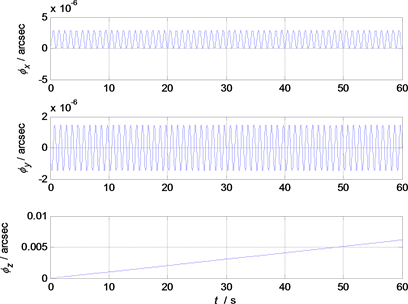Figure 1. Traditional algorithm attitude error of the two-sample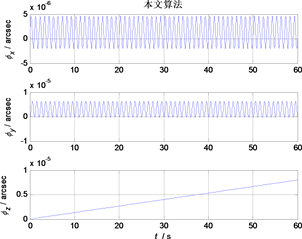Figure 2. Attitude error contrast of overlapping algorithm and algorithm of the two-sample in this paperFigure 3. Traditional algorithm attitude error of the three-sample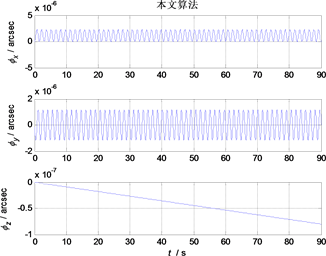Figure 4. Attitude error contrast of overlapping algorithm and algorithm of the three-sample in this paper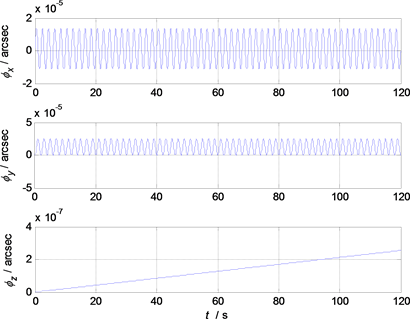Figure 5. Traditional algorithm attitude error of the four-sample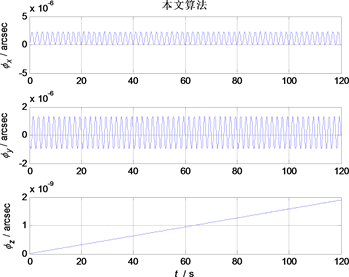Figure 6. Attitude error contrast of overlapping algorithm and algorithm of the four-sample in this paper

  李海涛, 曹咏弘, 祖静. 等效旋转矢量法在旋转弹姿态解算中的应用[J]. 测试技术学报, 2011, 25(4): 287-291.  程承, 潘泉, 李汉舟. 一种新的捷联惯导系统圆锥误差补偿算法研究[J]. 弹箭与制导学报, 2014, 34(1): 1-4.  Bortz, J.E. (2007) A New Mathematical Formulation for Strapdown Inertial Navigation. IEEE Trans-actions on Aerospace & Electronic Systems, AES-7, 61-66. https://doi.org/10.1109/TAES.1971.310252  Jordan, J.W. (1969) An Accurate Strapdown Direction Cosine Algorithm.  秦永元, 张士邈. 捷联惯导姿态更新的四子样旋转矢量优化算法研究[J]. 中国惯性技术学报, 2001, 9(4): 1-7.  陈建锋, 陈熙源, 祝雪芬. 一种基于二次优化的改进圆锥算法[J]. 中国惯性技术学报, 2012, 20(2): 131-135.  丁杨斌, 满顺强, 申功勋. 一种新的捷联惯导系统圆锥误差补偿算法[J]. 北京航空航天大学学报, 2007, 33(11): 1261-1263.  肖胜. 激光惯导系统流水式旋转矢量算法研究[J]. 光学与光电技术, 2015, 13(4): 51-56.  王真, 高凤岐, 高敏, 等. 旋转矢量多迭代捷联姿态解算误差补偿算法[J]. 中国测试, 2016, 42(8): 113-117.  杨浩天, 汪立新, 王琪, 等. 一种改进的重叠式三子样圆锥误差补偿算法[J]. 电光与控制, 2017(3): 20-23.